# Charge and Coulombs Law SPH 4 U Coulombs

• Slides: 28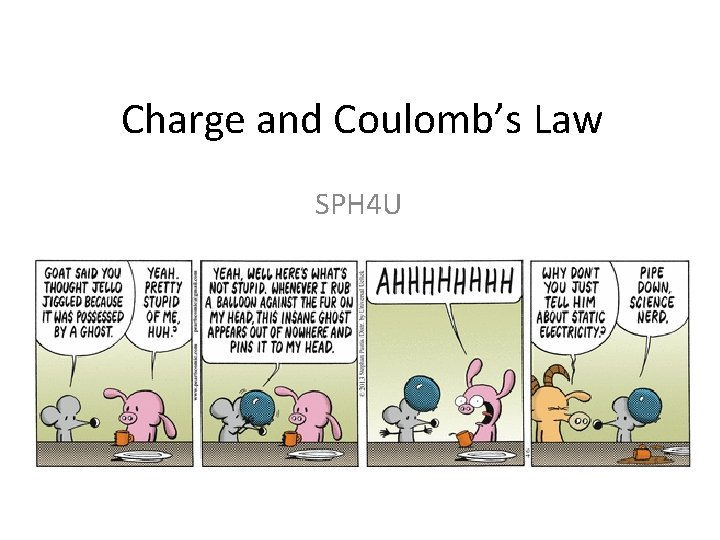Charge and Coulomb’s Law SPH 4 UCoulomb’s Law The magnitude of the electrostatic force between two charged objects is: the charges on objects 1 and 2, measured in Coulombs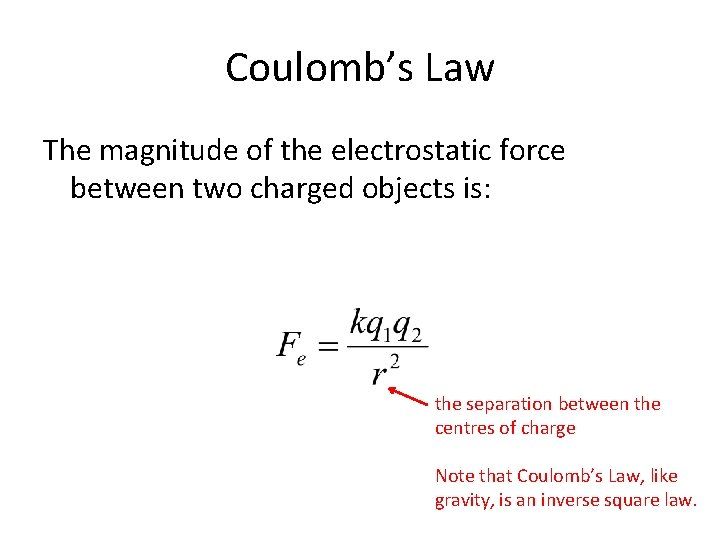Coulomb’s Law The magnitude of the electrostatic force between two charged objects is: the separation between the centres of charge Note that Coulomb’s Law, like gravity, is an inverse square law.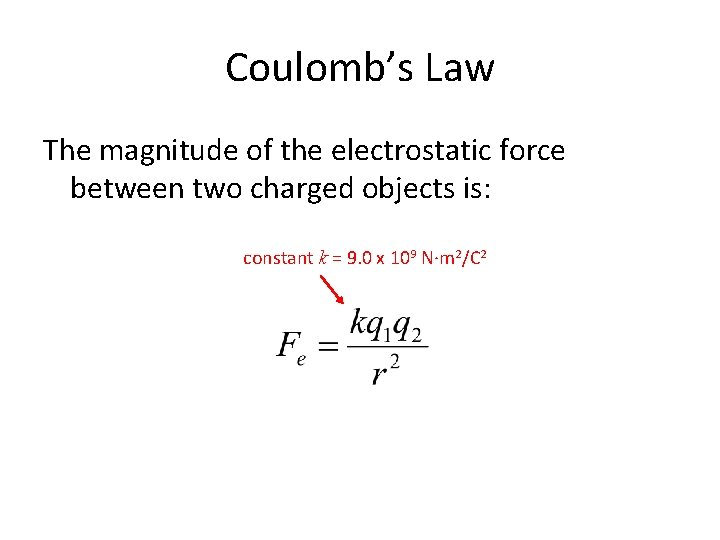Coulomb’s Law The magnitude of the electrostatic force between two charged objects is: constant k = 9. 0 x 109 N∙m 2/C 2The Law of Electric Charges The direction of the force is determined by the Law of Electric Charges: • Objects with unlike charge attract. • Objects with like charge repel. • Charged objects attract (some) neutral objects.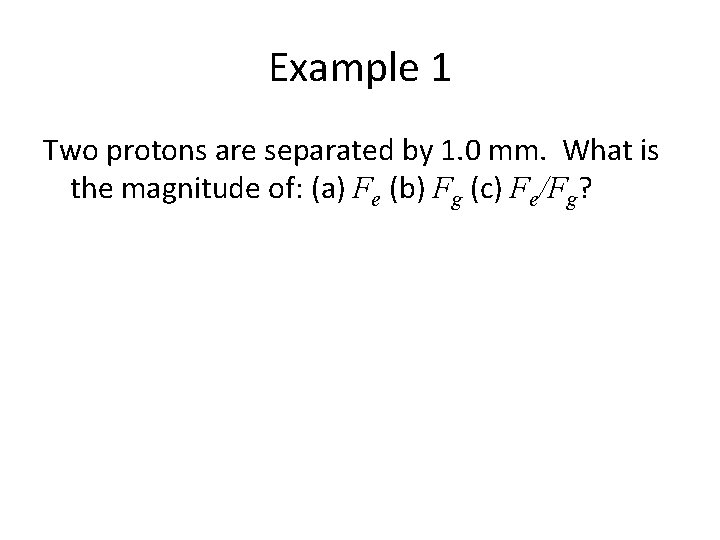Example 1 Two protons are separated by 1. 0 mm. What is the magnitude of: (a) Fe (b) Fg (c) Fe/Fg?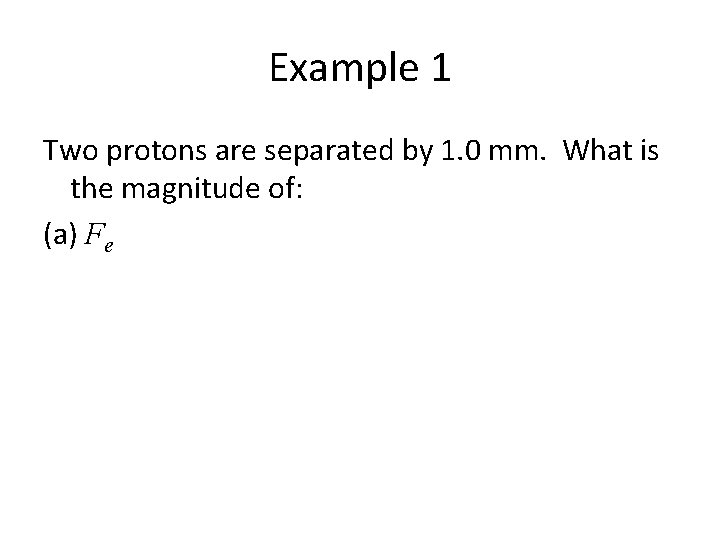Example 1 Two protons are separated by 1. 0 mm. What is the magnitude of: (a) Fe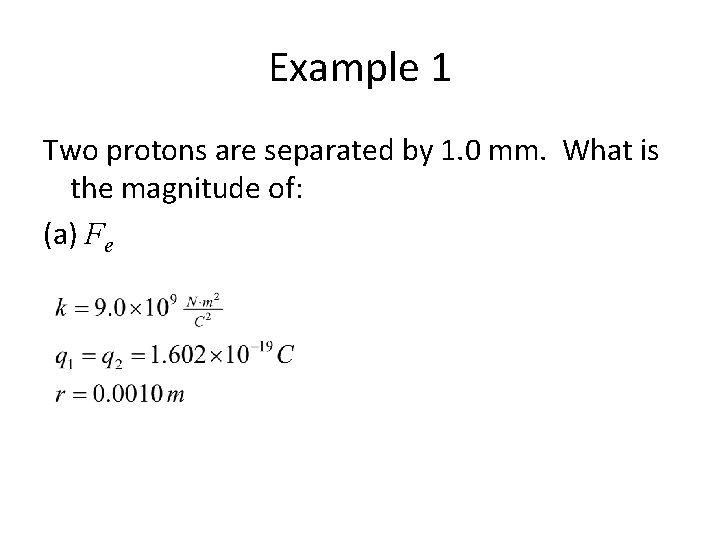Example 1 Two protons are separated by 1. 0 mm. What is the magnitude of: (a) Fe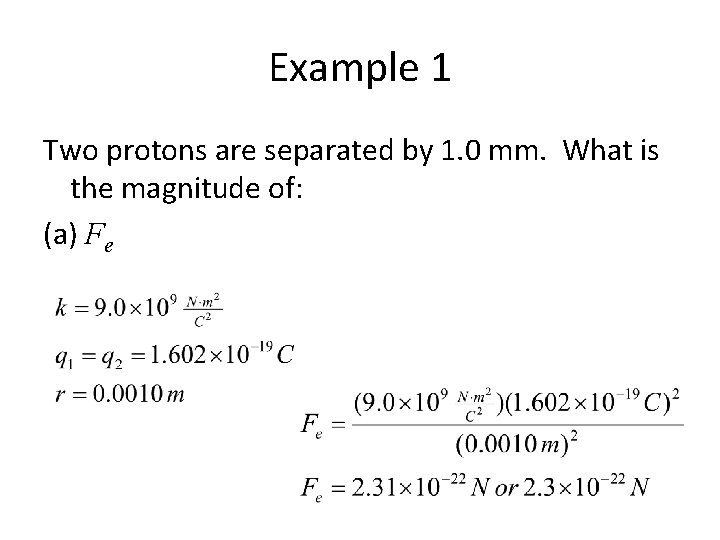Example 1 Two protons are separated by 1. 0 mm. What is the magnitude of: (a) FeExample 1 Two protons are separated by 1. 0 mm. What is the magnitude of: (a) Fe Note that this force will be repulsive.Example 1 Two protons are separated by 1. 0 mm. What is the magnitude of: (b) Fg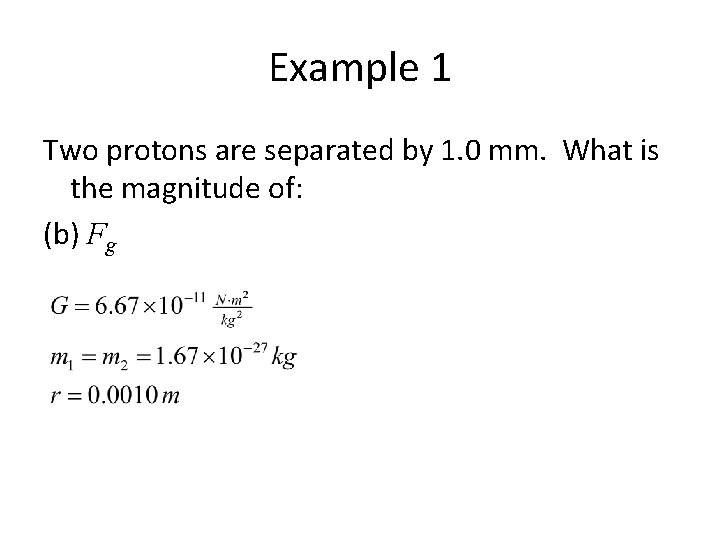Example 1 Two protons are separated by 1. 0 mm. What is the magnitude of: (b) Fg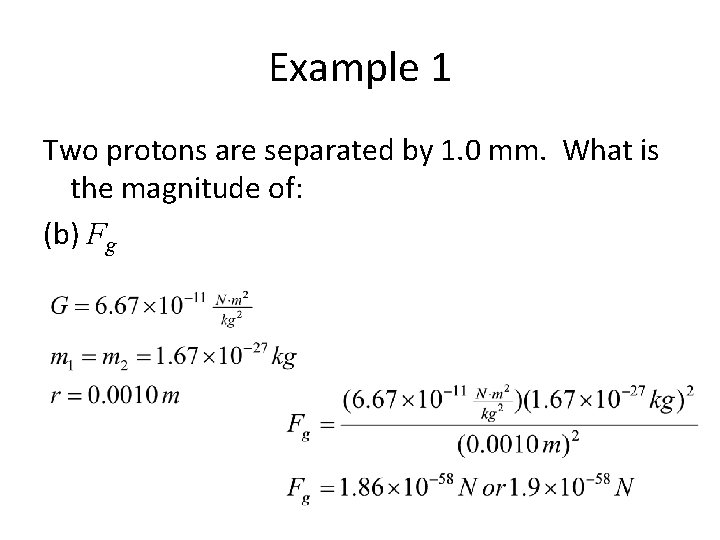Example 1 Two protons are separated by 1. 0 mm. What is the magnitude of: (b) Fg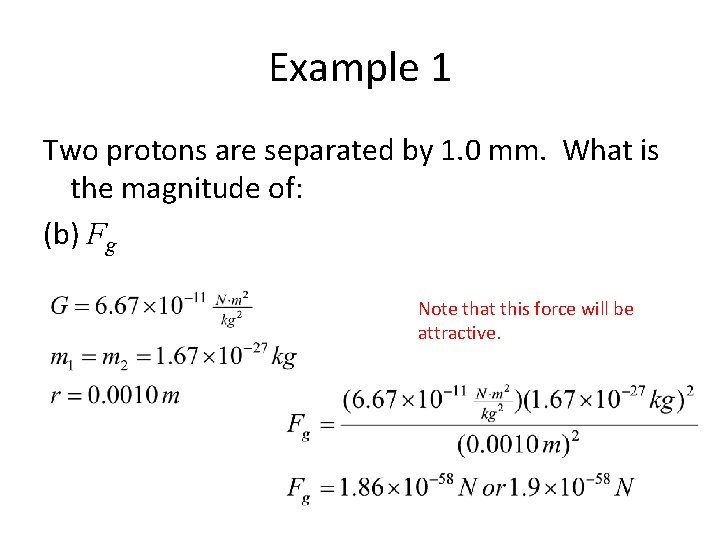Example 1 Two protons are separated by 1. 0 mm. What is the magnitude of: (b) Fg Note that this force will be attractive.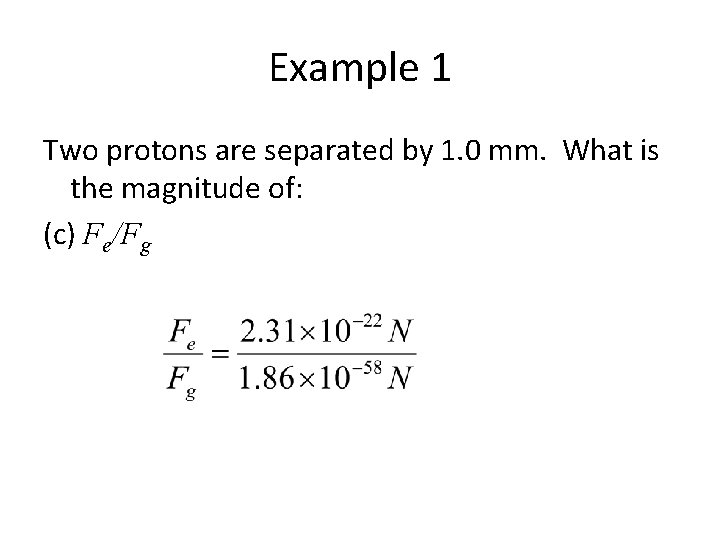Example 1 Two protons are separated by 1. 0 mm. What is the magnitude of: (c) Fe/Fg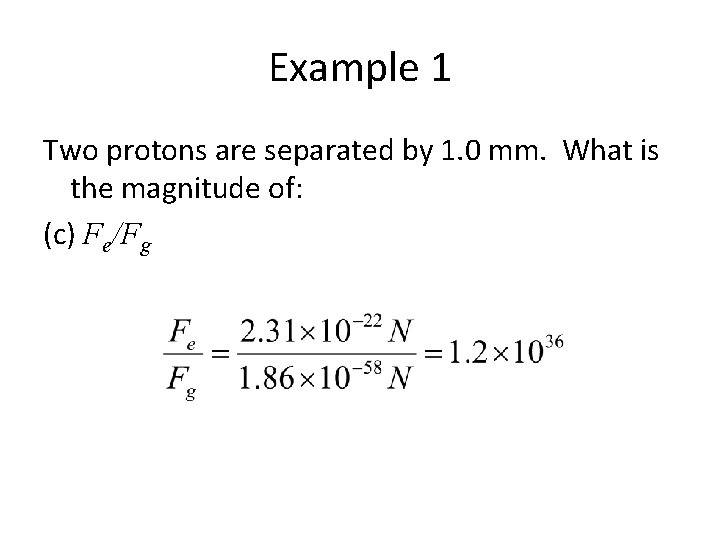Example 1 Two protons are separated by 1. 0 mm. What is the magnitude of: (c) Fe/Fg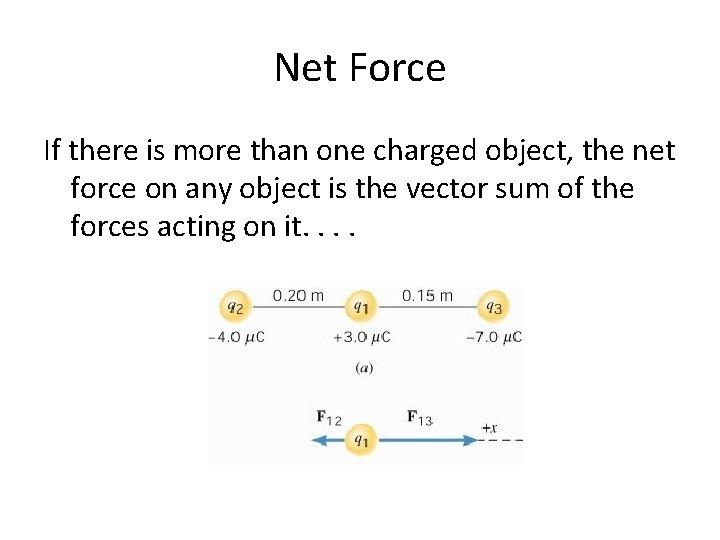Net Force If there is more than one charged object, the net force on any object is the vector sum of the forces acting on it. .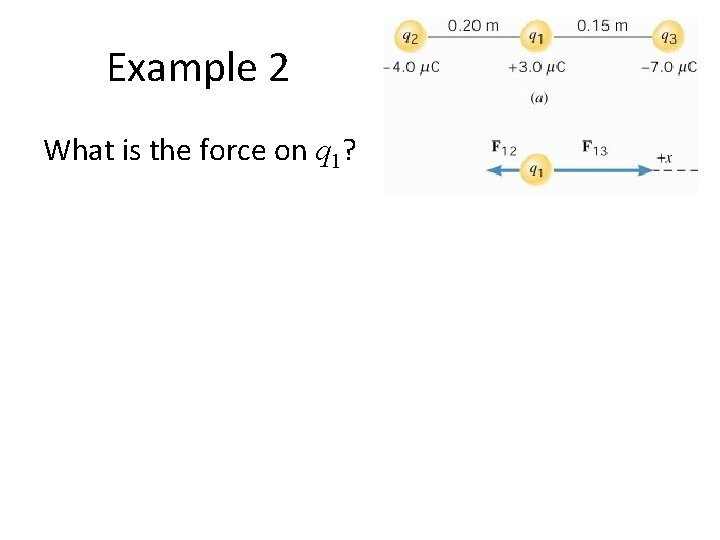Example 2 What is the force on q 1?Example 2 What is the force on q 1?Example 2 What is the force on q 1?Example 2 What is the force on q 1?Example 3 What is the force on the -0. 60 m. C charge? 6. 0 cmExample 3 What is the force on the -0. 60 m. C charge? 2 1 6. 0 cm 3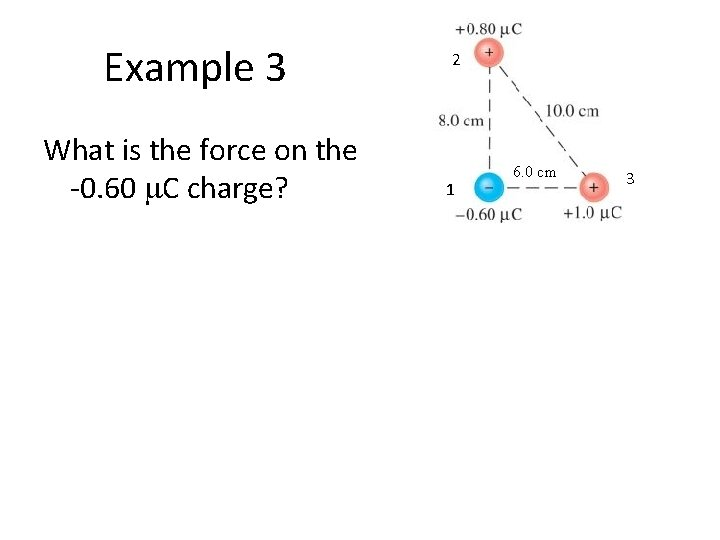Example 3 What is the force on the -0. 60 m. C charge? 2 1 6. 0 cm 3Example 3 What is the force on the -0. 60 m. C charge? 0. 675 N 1. 5 N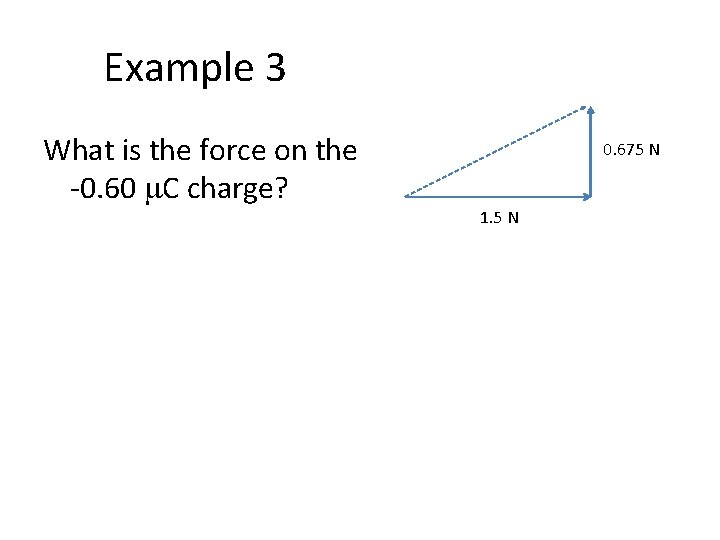Example 3 What is the force on the -0. 60 m. C charge? 0. 675 N 1. 5 NExample 3 What is the force on the -0. 60 m. C charge? 0. 675 N 1. 5 N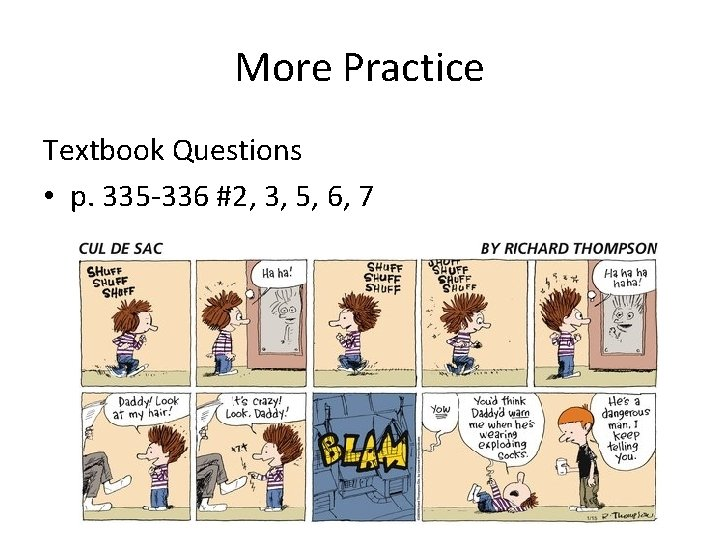More Practice Textbook Questions • p. 335 -336 #2, 3, 5, 6, 7